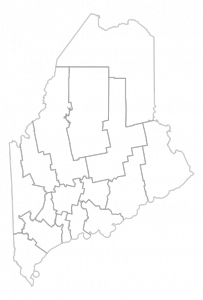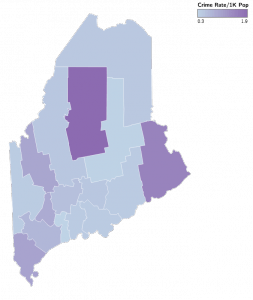Want to share your content on R-bloggers? click here if you have a blog, or here if you don't.

Even though it’s still at version `0.4`, the `ggvis` package has quite a bit of functionality and is highly useful for exploratory data analysis (EDA). I wanted to see how geographical visualizations would work under it, so I put together six examples that show how to use various features of `ggvis` for presenting static & interactive cartographic creations. Specifically, the combined exercises demonstrate:

• basic map creation
• basic maps with points/labels
• dynamic choropleths (with various scales & tooltips)
• applying projections and custom color fills (w/tooltips)
• apply projections and projecting coordinates for plotting (w/tooltips that handle missing data well)

If you want to skip the post and head straight to the code you can head on over to github, peruse the R markdown file on RPubs or play with the shiny version. You’ll need that code to actually run any of the snippets below since I’m leaving out some code-cruft for brevity. Also, all the map graphics below were generated by saving the `ggvis` output as PNG files (for best browser compatibility), right from the `ggvis` renderer popup. Click/tap each for a larger version.

### Basic PolygonsEven though we still need the help of `ggplot2`‘s `fortify`, it’s pretty straightforward to crank out a basic map in `ggvis`:

```maine <- readOGR("data/maine.geojson", "OGRGeoJSON")   map <- ggplot2::fortify(maine, region="name")   map %>% ggvis(~long, ~lat) %>% group_by(group, id) %>% layer_paths(strokeOpacity:=0.5, stroke:="#7f7f7f") %>% hide_legend("fill") %>% hide_axis("x") %>% hide_axis("y") %>% set_options(width=400, height=600, keep_aspect=TRUE)```

The code is very similar to one of the ways we render the same image in `ggplot`. We first read in the shapefile, convert it into a data frame we can use for plotting, group the polygons properly, render them with `layer_paths` and get rid of chart junk. Now, `ggvis` (to my knowledge as of this post) has no equivalent of `coord_map`, so we have to rely on the positioning in the projection and work out the proper `height` and `width` parameters to use with a uniform aspect ratio (`keep_aspect=TRUE`).

For those not familiar with `ggvis` the `~` operator lets us tell `ggivs` which columns (or expressions using columns) to map to function parameters and `:=` operator just tells it to use a raw, un-scaled value. You can find out more about why the tilde was chosen or about the various other special operators.

### Basic AnnotationsYou can annotate maps in an equally straightforward way.

```county_centers <- maine %>% gCentroid(byid=TRUE) %>% data.frame %>% cbind(name=maine\$name %>% gsub(" County, ME", "", .) )   map %>% group_by(group, id) %>% ggvis(~long, ~lat) %>% layer_paths(strokeWidth:=0.25, stroke:="#7f7f7f") %>% layer_points(data=county_centers, x=~x, y=~y, size:=8) %>% layer_text(data=county_centers, x=~x+0.05, y=~y, text:=~name, baseline:="middle", fontSize:=8) %>% hide_legend("fill") %>% hide_axis("x") %>% hide_axis("y") %>% set_options(width=400, height=600, keep_aspect=TRUE)```

Note that the `group_by` works both before or after the `ggvis` call. Consistent pipe idioms FTW!

Here, we’re making a data frame out of the county centroids and names then using that in a call to `layer_points` and `layer_text`. Note how you can change the data source for each layer (just like in `ggplot)` and use expressions just like in `ggplot` (we moved the text just slightly to the right of the dot).

Since `ggvis` outputs vega and uses D3 for rendering, you should probably take a peek at those frameworks as it will help you understand the parameter name differences between `ggvis` and `ggplot`.

### Basic ChoroplethsThere are actually two examples of this basic state choropleth in the code, but one just uses a different color scale, so I’ll just post the code for one here. This is also designed for interactivity (it has tooltips and lets you change the fill variable) so you should run it locally or look at the shiny version.

```"))}   map %>% group_by(group, id)%>% ggvis(~long, ~lat)%>% layer_paths(fill=input_select(label="Crime:", choices=crime_1k %>% select(ends_with("1k"))%>%colnames%>%sort, id="Crime", map=as.name), strokeWidth:=0.5, stroke:="white")%>% scale_numeric("fill", range=c("#bfd3e6", "#8c6bb1" ,"#4d004b"))%>% add_tooltip(crime_values, "hover")%>% add_legend("fill", title="Crime Rate/1K Pop")%>% hide_axis("x")%>% hide_axis("y")%>% set_options(width=400, height=600, keep_aspect=TRUE)# read in some crime & population data for maine counties me_pop <- read.csv("data/me_pop.csv", stringsAsFactors=FALSE) me_crime <- read.csv("data/me_crime.csv", stringsAsFactors=FALSE)   # get it into a form we can use (and only use 2013 data)   crime_1k <- me_crime %>% filter(year==2013) %>% select(1,5:12) %>% left_join(me_pop) %>% mutate(murder_1k=1000*(murder/population_2010), rape_1k=1000*(rape/population_2010), robbery_1k=1000*(robbery/population_2010), aggravated_assault_1k=1000*(aggravated_assault/population_2010), burglary_1k=1000*(burglary/population_2010), larceny_1k=1000*(larceny/population_2010), motor_vehicle_theft_1k=1000*(motor_vehicle_theft/population_2010), arson_1k=1000*(arson/population_2010))   # normalize the county names   map %<>% mutate(id=gsub(" County, ME", "", id)) %>% left_join(crime_1k, by=c("id"="county"))   # this is for the tooltip. it does a lookup into the crime data frame and # then uses those values for the popup   crime_values <- function(x) { if(is.null(x)) return(NULL) y <- me_crime %>% filter(year==2013, county==x\$id) %>% select(1,5:12) sprintf("%s", paste0("", names(y), ":", format(y), collapse=" ```

You can omit the `input_select` bit if you just want to do a single choropleth (just map `fill` to a single variable). The `input_select` tells `ggvis` to make a minimal bootstrap sidebar-layout scaffold around the actual graphic to enable variable interaction. In this case we let the user explore different types of crimes (by 1K population) and we also have a tooltip that shows the #’s of each crime in each county as we hover.

### Projections and Custom ColorsWe’re pretty much (mostly) re-creating a previous post in this example and making a projected U.S. map with drought data (as of 2014-12-23).

```"))}   map_d %>% group_by(group, id)%>% ggvis(~long, ~lat)%>% layer_paths(fill:=~fill_col, strokeOpacity :=0.5, strokeWidth :=0.25)%>% add_tooltip(drought_values, "hover")%>% hide_legend("fill")%>% hide_axis("x")%>% hide_axis("y")%>% set_options(width=900, height=600, keep_aspect=TRUE)us <- readOGR("data/us.geojson", "OGRGeoJSON") us <- us[!us\$STATEFP %in% c("02", "15", "72"),]   # same method to change the projection   us_aea <- spTransform(us, CRS("+proj=laea +lat_0=45 +lon_0=-100 +x_0=0 +y_0=0 +a=6370997 +b=6370997 +units=m +no_defs"))   map <- ggplot2::fortify(us_aea, region="GEOID")   droughts <- read.csv("data/dm_export_county_20141223.csv") droughts\$id <- sprintf("%05d", as.numeric(as.character(droughts\$FIPS))) droughts\$total <- with(droughts, (D0+D1+D2+D3+D4)/5)   map_d <- merge(map, droughts, all.x=TRUE)   # pre-make custom colors per county   ramp <- colorRampPalette(c("white", brewer.pal(n=9, name="YlOrRd")), space="Lab")   map_d\$fill_col <- as.character(cut(map_d\$total, seq(0,100,10), include.lowest=TRUE, labels=ramp(10))) map_d\$fill_col <- ifelse(is.na(map_d\$fill_col), "#FFFFFF", map_d\$fill_col)   drought_values <- function(x) { if(is.null(x) | !(x\$id %in% droughts\$id)) return(NULL) y <- droughts %>% filter(id==x\$id) %>% select(1,3,4,6:10) sprintf("%s", paste0("", names(y), ":", format(y), collapse=" ```

It’s really similar to the previous code (and you may/should be familiar with the Albers transform from the previous post).

### World Domination```world <- readOGR("data/ne_50m_admin_0_countries.geojson", layer="OGRGeoJSON") world <- world[!world\$iso_a3 %in% c("ATA"),] world <- spTransform(world, CRS("+proj=wintri"))   map_w <- ggplot2::fortify(world, region="iso_a3")   # really quick way to get coords from a KML file   launch_sites <- rbindlist(lapply(ogrListLayers("data/launch-sites.kml")[c language="(1:2,4:9)"][/c], function(layer) { tmp <- readOGR("data/launch-sites.kml", layer) places <- data.table(coordinates(tmp)[,1:2], as.character(tmp\$Name)) })) setnames(launch_sites, colnames(launch_sites), c("lon", "lat", "name"))   # now, project the coordinates we extracted   coordinates(launch_sites) <- ~lon+lat launch_sites <- as.data.frame(SpatialPointsDataFrame(spTransform( SpatialPoints(launch_sites, CRS("+proj=longlat")), CRS("+proj=wintri")), [email protected]data))   map_w %>% group_by(group, id) %>% ggvis(~long, ~lat) %>% layer_paths(fill:="#252525", stroke:="white", strokeOpacity:=0.5, strokeWidth:=0.25) %>% layer_points(data=launch_sites, x=~lon, y=~lat, fill:="#cb181d", stroke:="white", size:=25, fillOpacity:=0.5, strokeWidth:=0.25) %>% hide_legend("fill") %>% hide_axis("x") %>% hide_axis("y") %>% set_options(width=900, height=500, keep_aspect=TRUE)```

The main differences in this example are the re-projection of the data we’re using. I grabbed a KML file of rocket launch sites from Wikipedia and made it into a data frame then [re]project those points into Winkel-Tripel for use with Winkel-Tripel world map made at the beginning of the example. The `ggplot` `coord_map` handles these transforms for you, so until there’s a `ggvis` equivalent, you’ll need to do it this way (though, there’s not Winkel-Tripel projection in the `mapproject` package so you kinda need to do it this way for `ggplot` as well for this projection).

### Wrapping Up

There’s [code 1=””on”” 2=””github”” language=””up””][/code]/code for the “normal”, `Rmd` and Shiny versions of these examples. Give each a go and try tweaking various parameters, changing up the tooltips or using your own data. Don’t forget to drop a note in the comments with any of your creations and use github for any code issues.